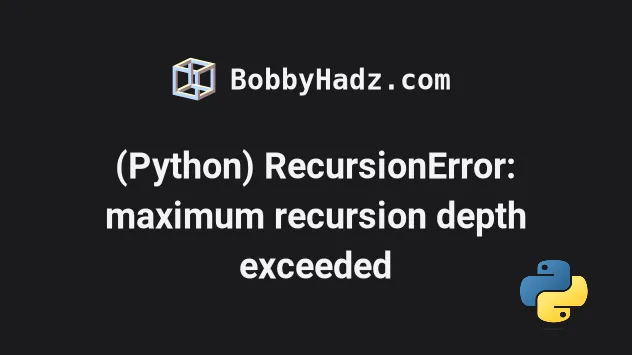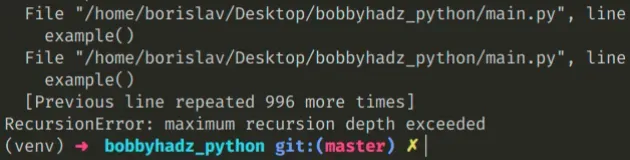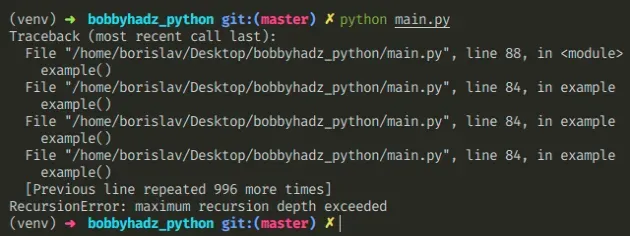# (Python) RecursionError: maximum recursion depth exceededLast updated: May 1, 2022Photo from Unsplash

## (Python) RecursionError: maximum recursion depth exceeded#

The Python "RecursionError: maximum recursion depth exceeded" occurs when a function is being called so many times that the invocations exceed the recursion limit. To solve the error, specify a base case that has to be met to exit the recursion or set a higher recursion limit.Here is an example of how the error occurs.

main.py
```Copied!```def example():
example()

# ⛔️ RecursionError: maximum recursion depth exceeded
example()
``````

We call the function, which then calls itself until the recursion limit is exceeded.

You can get the current value of the recursion limit by using the `sys.getrecursionlimit()` method.

main.py
```Copied!```import sys

# 👇️ 1000
print(sys.getrecursionlimit())

# 👇️ set recursion limit to 2000
sys.setrecursionlimit(2000)

# 👇️ 2000
print(sys.getrecursionlimit())
``````

The getrecursionlimit method returns the maximum depth of the Python interpreter stack.

You can use the setrecursionlimit method if you need to update this value.

To solve the error from the example, we have to specify a condition at which the function stops calling itself.

main.py
```Copied!```counter = 0

def example(num):
global counter

if num < 0:
return # 👈️ this stops the function from endlessly calling itself

counter += 1
example(num - 1)

example(3)

print(counter)  # 👉️ 4
``````

This time we check if the function was invoked with a number that is less than `0` on every invocation.

If the number is less than `0`, we simply return from the function so we don't exceed the maximum depth of the Python interpreter stack.

If the passed in value is not less than zero, we call the function with the passed in value minus `1`, which keeps us moving toward the case where the `if` check is satisfied.

A recursive function calls itself until a condition is met. If there is no condition to be met in your function, it will call itself until the maximum depth of the Python interpreter stack is exceeded.

You might also get this error if you have an infinite loop that calls a function somewhere.

ma
```Copied!```def do_math(a, b):
return a + b

while True:
result = do_math(10, 10)
print(result)
``````
Our `while` loop keeps calling the function and since we don't have a condition that would exit the loop, we eventually exceed the interpreter stack.

This works in a very similar way to a function calling itself without a base condition.

Here's an example of how to specify a condition that has to be met to exit the loop.

main.py
```Copied!```def do_math(a, b):
return a + b

total = 0
i = 10

while i > 0:
total += do_math(5, 5)

i = i - 1

print(total)  # 👉️ 100
``````

If the `i` variable is equal to or less than `0`, the condition in the `while` loop is not satisfied, so we exit the loop.

If you can't track exactly where your error occurs, look at the error message.The screenshot above shows that the error occurred on line `84` in the `example()` function.

You can also see that the error occurred in the `main.py` file.

## Conclusion#

The Python "RecursionError: maximum recursion depth exceeded" occurs when a function is being called so many times that the invocations exceed the recursion limit. To solve the error, specify a base case that has to be met to exit the recursion or set a higher recursion limit.

I wrote a book in which I share everything I know about how to become a better, more efficient programmer.You can use the search field on my Home Page to filter through all of my articles.